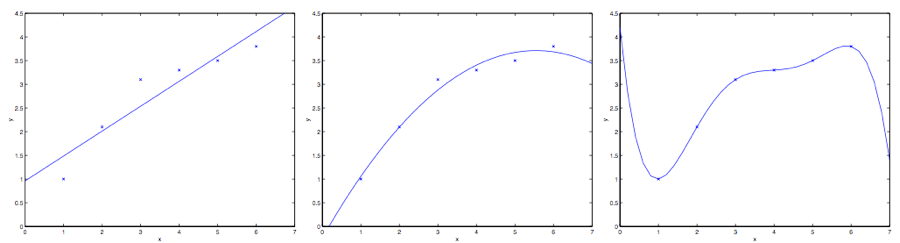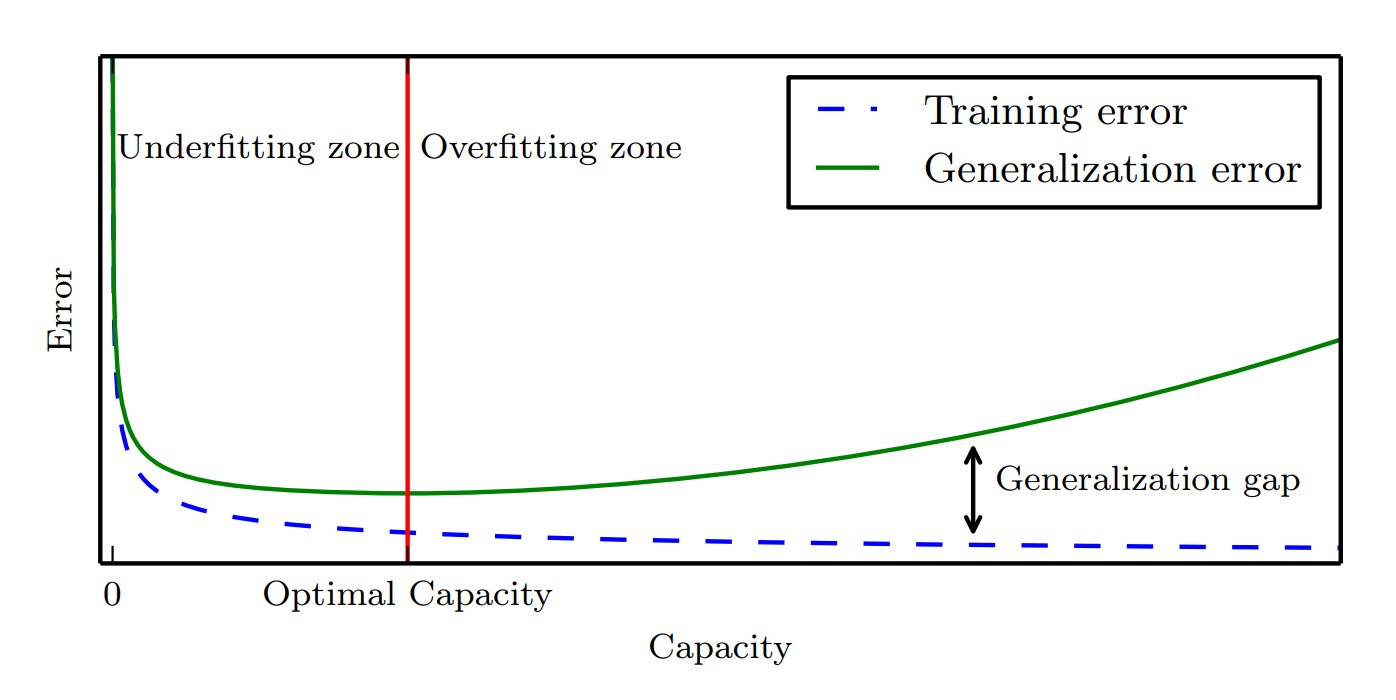# 模型容量、欠拟合和过拟合

$\frac{1}{2}(f(\boldsymbol{x}^{(i)train})-y^{(i)train})^2$

$\frac{1}{2}(f(\boldsymbol{x}^{(i)test})-y^{(i)test})^2$• 降低模型在训练集上的误差。
• 缩小训练集误差和测试集误差之间的差距。1. Andrew Ng：CS229 Lecture Notes
2. Ian Goodfellow and Yoshua Bengio and Aaron Courville: Deep Learning